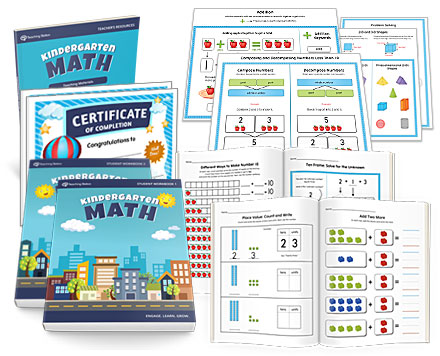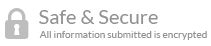• Teacher Approved

## Product ID: MTSMATH-KG-SET

 Regular Price \$59.99
 Now \$27.95
Color:### Product Description

The Complete Kindergarten Math Curriculum is a comprehensive, well-balanced set of workbooks that contain easy to understand activities and exercises to help students learn and master fundamental math concepts during the kindergarten year.

Filled with 683 attention-grabbing lessons, this easy-to-use curriculum includes colorfully illustrated activities and worksheets covering place value, skip counting, addition, subtraction, word problems, counting money, tally marks, two and three-dimensional shapes, reading time, and more.

The open-and-go format includes helpful teaching tools, a wide variety of engaging student activities, and fun yet practical worksheets that prove beneficial in a variety of settings.

The Complete Kindergarten Math Curriculum is part of the MTS Math Program.

### Part 1 Major Skills and Concepts

• Recognize numbers 0 to 100.
• Understand and identify number sequencing (before, between and after) up to 100.
• Recognize ordinal position and numbers.
• Count by 1s to 100 while understanding the relationship a number has with one another (e.g., the number "50" is greater than "20" and less than "100").
• Identify basic shapes including squares, circles, triangles, and rectangles.
• Demonstrate correct number formation using the continuous stroke method.
• Gain a strong foundation for place value by working with tens and ones.
• Understand math symbols (e.g., plus sign and equal sign) along with math expressions (e.g., 1 + 2 ).
• Develop the conceptual understanding of addition - creating new numbers.
• Learn to add numbers within 10 using pictures, a number line, and other visual counters.
• Develop an attention to detail, precision, and accuracy through number sense activities.
• Learn to count by 2s and 10s.

### Part 2 Major Skills and Concepts

• Learn to compose and decompose numbers within 10 (e.g., 4 + 3 = 7 and 7 = 4 + 3).
• Develop number sense by the use of numbers in different ways and situations.
• Make sense of word problems and develop the skill to solve for the unknown number.
• Learn and apply math rules to solve basic addition and subtraction problems.
• Understand and solve number sentences (e.g., 2 + 3 = 5 ) within the context of 10.
• Apply different techniques to comprehend, interpret, evaluate, and solve math problems.
• Learn to count by 5s.
• Learn and use tally marks to quickly count by 5s and record ongoing results.
• Identify and describe two-dimensional shapes including pentagon, hexagon, parallelogram, and trapezoid.
• Identify and decompose two-dimensional shapes.
• Develop a basic understanding of geometric terms such as side, vertex (corners) and parallel lines.
• Develop the conceptual understanding of subtraction as taking apart (or taking from) a number.
• Learn to subtract numbers within 10 using pictures, a ten-frame, and other visual counters.
• Recognize pennies, nickels, dimes, quarters and identify their values.
• Understand how to count money using coins.
• Recognize three-dimensional shapes including sphere, cube, cylinder, pyramid, cone, triangular prism, and rectangular prism.
• Understand the difference between two and three-dimensional shapes.
• Understand how time is measured, how many hours are in a day and how many minutes are in an hour.
• Read time using a digital and analog clock.
• Learn the days of the week, months of the year and their ordinal sequence.

### What is Included

• Student Workbook Part 1 and Student Workbook Part 2 containing 25 units total with two additional review units (one at the end of each book).
• Teacher Resource Book containing tools to make teaching simple and easy-to-understand (including flashcards and concept description posters).
• Easy to follow instructions for worksheets and suggestions for implementing the program activities.
• Answer keys for applicable worksheets.
• Modern, eye-catching graphics designed for an elementary student.
• Fun and practical activities including puzzles, mazes, and crafts.
• Bonus Materials: Achievement award.

### Can I see a sample of the program lessons?

3 rating(s)
Current rating: 4.7 out of 5 stars
 *Public Name This will be the nickname shown on your review. *Email Your email will not be published. *Your Rating 1 2 3 4 5 Your Review
By Helen Marissa
on Jun 27, 2017

By Carol
on May 23, 2017

By Julani
on May 17, 2017
Easy to use, thorough and interesting. The kids love it.

#### File Info

 Package: 5 Files Size: 1,051 Total Pages Format: PDF